SPSS TUTORIALS BASICS ANOVA REGRESSION FACTOR CORRELATION

# Spearman Rank Correlations – Simple Tutorial

A Spearman rank correlation is a number between -1 and +1 that indicates to what extent 2 variables are monotonously related.

## Spearman Correlation - Example

A sample of 1,000 companies were asked about their number of employees and their revenue over 2018. For making these questions easier, they were offered answer categories. After completing the data collection, the contingency table below shows the results.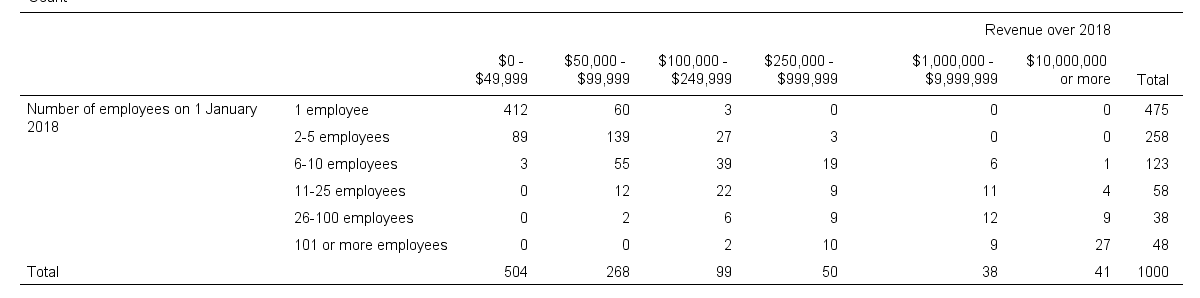The question we'd like to answer is is company size related to revenue? A good look at our contingency table shows the obvious: companies having more employees typically make more revenue. But note that this relation is not perfect: there's 60 companies with 1 employee making $50,000 -$99,999 while there's 89 companies with 2-5 employees making $0 -$49,999. This relation becomes clear if we visualize our results in the chart below.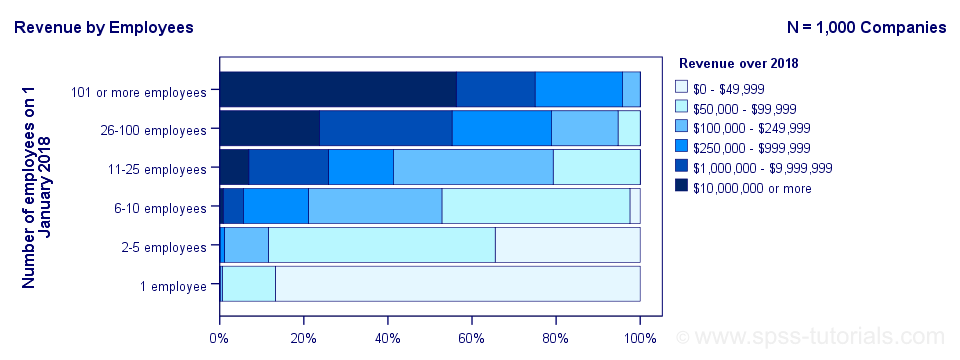The chart shows an undisputable positive monotonous relation between size and revenue: larger companies tend to make more revenue than smaller companies. Next question. How strong is the relation? The first option that comes to mind is computing the Pearson correlation between company size and revenue. However, that's not going to work because we don't have company size or revenue in our data. We only have size and revenue categories. Company size and revenue are ordinal variables in our data: we know that 2-5 employees is larger than 1 employee but we don't know how much larger.
So which numbers can we use to calculate how strongly ordinal variables are related? Well, we can assign ranks to our categories as shown below.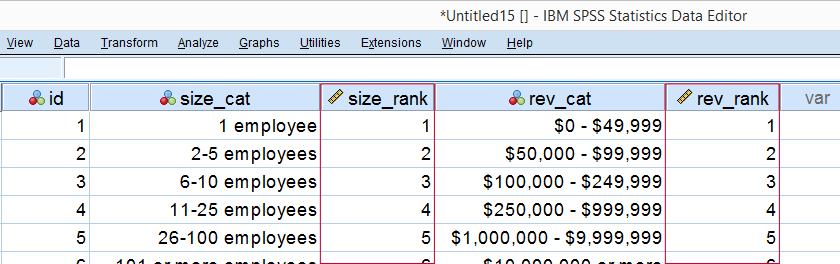As a last step, we simply compute the Pearson correlation between the size and revenue ranks. This results in a Spearman rank correlation (Rs) = 0.81. This tells us that our variables are strongly monotonously related. But in contrast to a normal Pearson correlation, we do not know if the relation is linear to any extent.

## Spearman Rank Correlation - Basic Properties

Like we just saw, a Spearman correlation is simply a Pearson correlation computed on ranks instead of data values or categories. This results in the following basic properties:

• Spearman correlations are always between -1 and +1;
• Spearman correlations are suitable for all but nominal variables. However, when both variables are either metric or dichotomous, Pearson correlations are usually the better choice;
• Spearman correlations indicate monotonous -rather than linear- relations;
• Spearman correlations are hardly affected by outliers. However, outliers should be excluded from analyses instead of determine whether Spearman or Pearson correlations are preferable;
• Spearman correlations serve the exact same purposes as Kendall’s tau.

## Spearman Rank Correlation - Assumptions

• The Spearman correlation itself only assumes that both variables are at least ordinal variables. This excludes all but nominal variables.
• The statistical significance test for a Spearman correlation assumes independent observations or -precisely- independent and identically distributed variables.

## Spearman Correlation - Example II

A company needs to determine the expiration date for milk. They therefore take a tiny drop each hour and analyze the number of bacteria it contains. The results are shown below.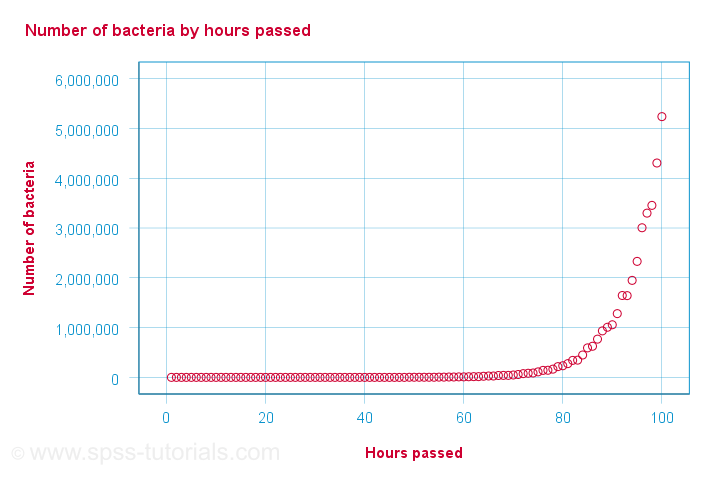For bacteria versus time,

• the Pearson correlation is 0.58 but
• the Spearman correlation is 1.00.

There is a perfect monotonous relation between time and bacteria: with each hour passed, the number of bacteria grows. However, the relation is very non linear as shown by the Pearson correlation.
This example nicely illustrates the difference between these correlations. However, I'd argue against reporting a Spearman correlation here. Instead, model this curvilinear relation with a (probably exponential) function. This'll probably predict the number of bacteria with pinpoint precision.

## Spearman Correlation - Formulas and Calculation

First off, an example calculation, exact significance levels and critical values are given in this Googlesheet (shown below).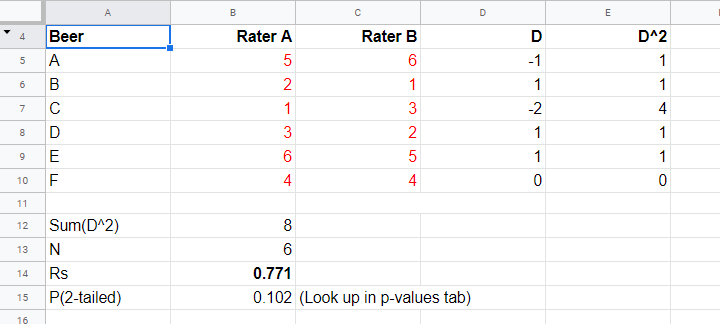Right. Now, computing Spearman’s rank correlation always starts off with replacing scores by their ranks (use mean ranks for ties). Spearman’s correlation is now computed as the Pearson correlation over the (mean) ranks.

Alternatively, compute Spearman correlations with $$R_s = 1 - \frac{6\cdot \Sigma \;D^2}{n^3 - n}$$
where $$D$$ denotes the difference between the 2 ranks for each observation.

For reasonable sample sizes of N ≥ 30, the (approximate) statistical significance uses the t distribution. In this case, the test statistic $$T = \frac{R_s \cdot \sqrt{N - 2}}{\sqrt{1 - R^2_s}}$$
follows a t-distribution with $$Df = N - 2$$
degrees of freedom.

This approximation is inaccurate for smaller sample sizes of N < 30. In this case, look up the (exact) significance level from the table given in this Googlesheet. These exact p-values are based on a permutation test that we may discuss some other time. Or not.

## Spearman Rank Correlation - Software

Spearman correlations can be computed in Googlesheets or Excel but statistical software is a much easier option. JASP -which is freely downloadable- comes up with the correct Spearman correlation and its significance level as shown below.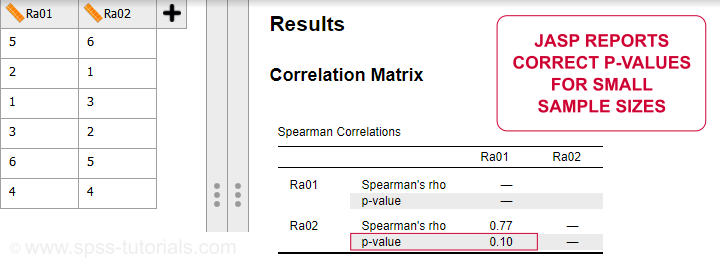SPSS also comes up with the correct correlation. However, its significance level is based on the t-distribution: $$t = \frac{0.77\cdot\sqrt{4}}{\sqrt{(1 - 0.77^2)}} = 2.42$$
and $$t(4) = 2.42,\;p = 0.072$$
Again, this approximation is only accurate for larger sample sizes of N ≥ 30. For N = 6, it is wildly off as shown below.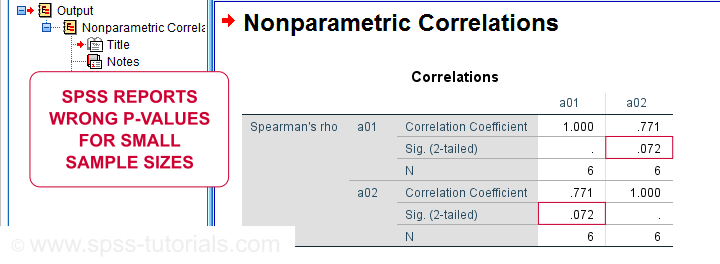# Tell us what you think!

*Required field. Your comment will show up after approval from a moderator.

# THIS TUTORIAL HAS 8 COMMENTS:

•### By Faria on September 12th, 2022

It's very intéressant. Thank you very much

•### By Jon K Peck on September 12th, 2022

The correlations procedure in SPSS also offers bootstrapped CI's and significance measures. In addition, the SPSSINC HETCOR extension command available from the Extension Hub offers polyserial and polychoric correlations that work for nominal, ordinal, and scale variables. The correlation type is determined automatically by the declared measurement levels of the variables.

•### By Ruben Geert van den Berg on September 13th, 2022

Hi Jon!

I installed and tested HETCOR but it throws quite some warnings such as:

Warning messages:
1: In FUN(X[[i]], ...) :
polychoric correlation between variables ordered.dta...1.. and ordered.dta...2.. produced warnings:
NaNs produced
NaNs produced...

I guess these merely mean that there's missing values in most variables? Why should the user receive warnings for this? I mean, "normal" SPSS procedures don't show warnings for missing values either, right?

Second, polychoric correlations are not suitable for variable pairs including a nominal variable, are they?

Last, when/why would you prefer polychoric correlations over Spearman/Kendall’s tau?

Or the same for polyserial correlations versus eta (as in the square root of eta squared)?

I mostly stumbled upon polyserial correlations for LISREL (ancient and long gone software for SEM, just like AMOS). In this case, the "end game" was CFA.

•### By Jon K Peck on September 13th, 2022

I have no control over what happens inside an R module used by an extension command, but I try to anticipate problematic input and issue a clearer error message than the typical R module provides. In the case of HETCOR, which uses the R hetcor module, that procedure sometimes fails in a way that can't be anticipated when it can't find a latent normality construct. The NANs usually come from convergence problems in its algorithm rather than being related to missing data.

hetcor computes a heterogenous correlation matrix, consisting of Pearson product-moment correlations between numeric variables, polyserial correlations between numeric and ordinal variables, and polychoric correlations between ordinal variables. For nominal variables, this would only be applicable when they are dichotomies.

If you can send me data and syntax, I'll see if I can find a reason for the failure in that case.

For polyserial correlation, the procedure tries to find a latent underlying bivariate normal distribution involving the ordinal variables.
Here's a quote from Wikipedia for polychoric.

This technique is frequently applied when analysing items on self-report instruments such as personality tests and surveys that often use rating scales with a small number of response options (e.g., strongly disagree to strongly agree). The smaller the number of response categories, the more a correlation between latent continuous variables will tend to be attenuated. Lee, Poon & Bentler (1995) have recommended a two-step approach to factor analysis for assessing the factor structure of tests involving ordinally measured items. Kiwanuka and colleagues (2022) have also illustrated the application of polychoric correlations and polychoric confirmatory factor analysis in nursing science. This aims to reduce the effect of statistical artifacts, such as the number of response scales or skewness of variables leading to items grouping together in factors. In some disciplines, the statistical technique is rarely applied however, some scholars  have demonstrated how it can be used as an alternative to the Pearson correlation.

•### By YY on September 14th, 2022

“Spearman correlation is simply a Pearson correlation computed on ranks”.

Is the normality assumption required theoretically for the ranks when we test the Spearman’s correlation coefficient using the t test?